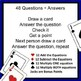# Solving Systems: Elimination Method: EDITABLE 8.EE.C.8, HSA.REI.C.5, MA.8.9.ASubject
Resource Type
File Type

Zip

(1 MB|20 pages)
Standards
Also included in:
1. This is a great addition to any classroom! All of these resources engage the students and can be inserted into your unit plan seamlessly! Topics are:Adding Fractions & Mixed Numbers 5.NF.A.1 { EDITABLE } 48 Questions GAMEDividing Fractions {48 Questions Game} EDITABLE 6.NS.A.1 {Mixed Numbers al
\$24.95
\$15.99
Save \$8.96
• Product Description
• StandardsNEW

A rigorous 60 MINUTE activity. HASSLE-FREE Math Game { Students Self Check Answers }

{ Distance Learning Friendly }

This game has 48 questions, covering all aspects of solving by elimination. It is easily differentiated since there are 4 levels of questions, as well it is editable, so you can tailor it for your class.

This resource covers the following concepts related to Elimination Method:

♥ Elimination method by Adding the 2 equations (no multiplication required)

♦ Elimination method by Subtracting the 2 equations (no multiplication required)

♣ Elimination method but students must multiply one equation by a constant

♠ Elimination method but students must multiply both equations by a constant

----------------------------------------------------------------------------------------------------------------------------------------------------------------

Top 3 Reasons to Use this Editable Game in the Classroom:

1. Hassle-Free: All of the instructions are student-friendly and set out in an easy to follow format. The students self-check their work, so the students work independently. This resource has been classroom tested and has proven to be an effective method for reviewing this topic.

2. Differentiate: this resource is easy to differentiate since it is divided into 4 categories of difficulty, so the students who need more practice in a certain area, can select the group of questions that will benefit them the most, also, this is EDITABLE, so the teacher can tailor the questions to the class needs.

3. Variation: This game introduces variation since there are 48 questions to pick from and the students randomly answer questions, so the game is not static.

Package includes:

* Rationale: how to use the product in the classroom

* Student Instruction handout

* Practical Tips for Teachers

* Game Booklet includes:

♥ 12 questions: elimination by adding 2 equations

♦ 12 questions: elimination by subtracting 2 equations

♣ 12 questions: elimination by multiplying ONE equation by a factor

♠ 12 questions: elimination by multiplying BOTH equations by a factor

* Answer key for all 48 questions

* 2 blank student response sheets

* pdf with all of the above contents

* EDITABLE 48 question game PowerPoint and rtf format

----------------------------------------------------------------------------------------------------------------------------------------------------------------

Can be used as:

* Math Lab

* Game for 1, 2, 3 or 4 players

* Peer Tutoring Game

* Homeschooling

* Stations

* Worksheets

* Differentiation

* Early Finishers

* Milestones Review

* Math Intervention

* Student Activity when you are away from the Classroom!

----------------------------------------------------------------------------------------------------------------------------------------------------------------

This is a math game in which one pair of students competes against another. The first team selects a card from a deck of cards and answers the matching question on the activity sheet (example 4 of hearts). The 2 students work cooperatively to obtain an answer. If correct they get a point, if not the other team can get the rebound point. Then team 2 selects a card, discusses the answer and so on. It is cooperative because the 2 students work together, yet competitive because it pits one team against another. The students have fun and get the math work done. As well, the students can work on this activity alone, can be used as a review.

You might also like:

I often provide { FREEBIES } and { PRODUCT UPDATES } to my followers!

Thank you for visiting :)

MatheMatters by Jacquie

===============================================

{ HOW TO GET PRICE REDUCTIONS ON FUTURE PRODUCTS }

* access your "My Purchases" page

* look for the "Provide Feedback" button

* click it

* give a rating

* TpT gives you "feedback credits"

* use credits to lower the cost of your future purchases.

* it helps me to determine which products are most valuable for you

* hope to hear from you soon!

Solve systems of linear equations exactly and approximately (e.g., with graphs), focusing on pairs of linear equations in two variables.
Prove that, given a system of two equations in two variables, replacing one equation by the sum of that equation and a multiple of the other produces a system with the same solutions.
Solve systems of two linear equations in two variables algebraically, and estimate solutions by graphing the equations. Solve simple cases by inspection. For example, 3𝘹 + 2𝘺 = 5 and 3𝘹 + 2𝘺 = 6 have no solution because 3𝘹 + 2𝘺 cannot simultaneously be 5 and 6.
Understand that solutions to a system of two linear equations in two variables correspond to points of intersection of their graphs, because points of intersection satisfy both equations simultaneously.
Analyze and solve pairs of simultaneous linear equations.
Total Pages
20 pages
Included
Teaching Duration
90 minutes
Report this Resource to TpT
Reported resources will be reviewed by our team. Report this resource to let us know if this resource violates TpT’s content guidelines.\$3.99
List Price:
\$4.99
You Save:
\$1.00